Courses

# Operational Amplifier

## 10 Questions MCQ Test Topicwise Question Bank for Electronics Engineering | Operational Amplifier

Description
This mock test of Operational Amplifier for Electronics and Communication Engineering (ECE) helps you for every Electronics and Communication Engineering (ECE) entrance exam. This contains 10 Multiple Choice Questions for Electronics and Communication Engineering (ECE) Operational Amplifier (mcq) to study with solutions a complete question bank. The solved questions answers in this Operational Amplifier quiz give you a good mix of easy questions and tough questions. Electronics and Communication Engineering (ECE) students definitely take this Operational Amplifier exercise for a better result in the exam. You can find other Operational Amplifier extra questions, long questions & short questions for Electronics and Communication Engineering (ECE) on EduRev as well by searching above.
QUESTION: 1

Solution:
QUESTION: 2

### The op-amp circuit shown below is initially nulled having a supply voltages of ± 15 V. The output voltage VQ is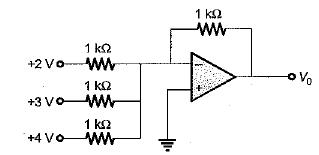Solution:

Given circuit represents an inverting summer using op-amp
Here,

R = R1 = R2 = R3 = Rf = 1 kΩ

and

V1 = +2 V, V2 = +3 V, V3 = +4 V

The output voltage is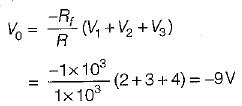QUESTION: 3

### For the circuit shown below, the output voltage is given by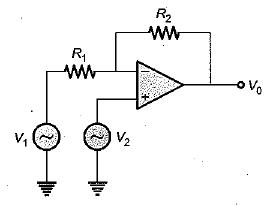Solution:

Using superposition theorem, the output voltage is given by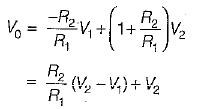QUESTION: 4

For an astabie multivibrator shown below,

R1 = 116 KΩ, R2 = 100 KΩ, and ± Vsat = ± 14 V.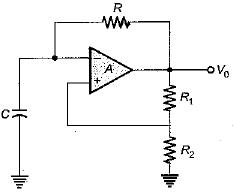The time constant of the circuit to produce an output of frequency 1 kHz will be

Solution:

The time period of the given a stablem ultivibrator circuit is given by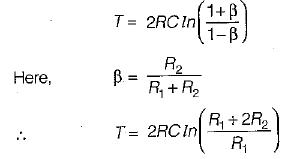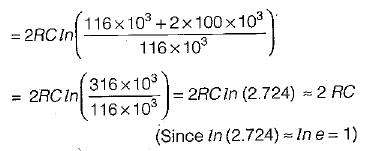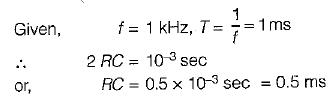QUESTION: 5

An ideal operational amplifier has input impedance of

Solution:

For an ideal OPAMP, input impedance is infinite while output impedance is zero.

QUESTION: 6

For the op-amp circuit shown below, R1 = 1 kΩ and Rf = 10 kΩ. The feedback factor β of this amplifier is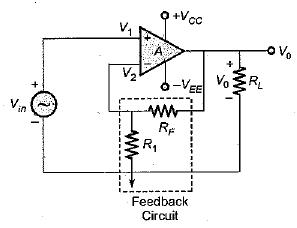Solution:

Given op -amp circuit re p re se n ts a c losed -loop non -inverting amplifier. The feed back factor is given by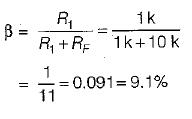QUESTION: 7

The order of input resistance in 741 OP-AMP is

Solution:

For a practical OPAMP in put impedance is of the order of 106Ω

QUESTION: 8

For a given op-amp, CMMR = 105 and differential gain Ad = 105. The common-mode gain of the op-amp is

Solution: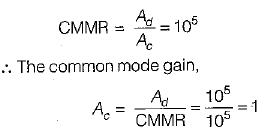QUESTION: 9

An inverting amplifier using the 741C must have a fiat response upto 40 kHz. The gain of the amplifier is 10 and slew rate is 0.5 V/μS. The maximum peak-to-peak input signal that can be applied without distorting the output is approximately equal to

Solution:

We know that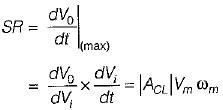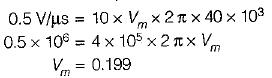QUESTION: 10

An idea! operational amplifier has
1. non-zero, off set voltage.
2. characteristics which do not drift with temperature.
3. an infinite voltage gain.
4. a CMMR more than 120 dB.
5. infinite bandwidth.
Of these statements:

Solution:

An ideal operational amplifier has

• zero offset voltage
• characteristics which do not drift with temperature
• infinite voltage gain
• infinite CMMR
• infinite bandwidth

Hence, statements 2 , 3 and 5 are only correct.